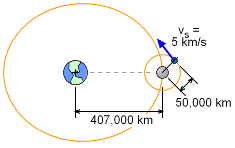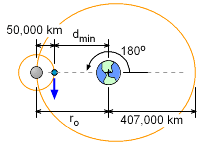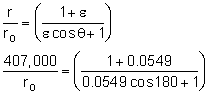Ch 2. Particle Force and Acceleration Multimedia Engineering Dynamics Rect.Coord. Normal/Tang. Coord. PolarCoord. Orbital Mechanics Computational Mechanics
 Chapter - Particle - 1. General Motion 2. Force & Accel. 3. Energy 4. Momentum - Rigid Body - 5. General Motion 6. Force & Accel. 7. Energy 8. Momentum 9. 3-D Motion 10. Vibrations Appendix Basic Math Units Basic Equations Sections Search eBooks Dynamics Fluids Math Mechanics Statics Thermodynamics Author(s): Kurt Gramoll ©Kurt GramollDYNAMICS - EXAMPLEMoon Satellite Example The moon orbits the earth with an eccentricity of 0.0549. The maximum distance between the moon and earth centers is 407,000 km. A small satellite also orbits the moon in a circular orbit with a velocity of 5 km/s at an elevation of 50,000 km. What is the minimum distance between the earth center and the satellite? Neglect any effect the earth has on the satellite. Both the moon and satellite move in the same plane. SolutionMoon and Satellite Orbits First, find the perigee distance, ro.407,000 = ro (1.0549 / 0.9451)    ro = 364,600 km Minimum distance    dmin = ro - 50,000 =364,600 - 50,000 dmin= 314,600 km

Practice Homework and Test problems now available in the 'Eng Dynamics' mobile app
Includes over 400 problems with complete detailed solutions.
Available now at the Google Play Store and Apple App Store.